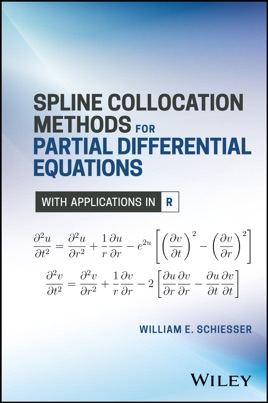• \$104.99

## Publisher Description

A comprehensive approach to numerical partial differential equations

Spline Collocation Methods for Partial Differential Equations combines the collocation analysis of partial differential equations (PDEs) with the method of lines (MOL) in order to simplify the solution process. Using a series of example applications, the author delineates the main features of the approach in detail, including an established mathematical framework. The book also clearly demonstrates that spline collocation can offer a comprehensive method for numerical integration of PDEs when it is used with the MOL in which spatial (boundary value) derivatives are approximated with splines, including the boundary conditions.

R, an open-source scientific programming system, is used throughout for programming the PDEs and numerical algorithms, and each section of code is clearly explained. As a result, readers gain a complete picture of the model and its computer implementation without having to fill in the details of the numerical analysis, algorithms, or programming. The presentation is not heavily mathematical, and in place of theorems and proofs, detailed example applications are provided.

Appropriate for scientists, engineers, and applied mathematicians, Spline Collocation Methods for Partial Differential Equations:
Introduces numerical methods by first presenting basic examples followed by more complicated applications Employs R to illustrate accurate and efficient solutions of the PDE models Presents spline collocation as a comprehensive approach to the numerical integration of PDEs and an effective alternative to other, well established methods Discusses how to reproduce and extend the presented numerical solutions Identifies the use of selected algorithms, such as the solution of nonlinear equations and banded or sparse matrix processing Features a companion website that provides the related R routines
Spline Collocation Methods for Partial Differential Equations is a valuable reference and/or self-study guide for academics, researchers, and practitioners in applied mathematics and engineering, as well as for advanced undergraduates and graduate-level students.